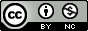Note: This document has been updated for pomp version 2.

Produced in R version 4.0.2 using pomp version 3.1.1.0.

## Partially observed Markov process (POMP) models

• Data \(y^*_1,\dots,y^*_N\) collected at times \(t_1<\dots<t_N\) are modeled as noisy, incomplete, and indirect observations of a Markov process \(\{X(t), t\ge t_0\}\).

• This is a partially observed Markov process (POMP) model, also known as a hidden Markov model or a state space model.

• The POMP class of models can accommodate a variety of complexities that commonly arise, especially in biological models:
• latent variables
• nonlinear dynamics
• non-Gaussian distributions
• continuous-time models (as well as discrete-time models)
• intractable likelihoods
• non-differentiable models

## Goals

The R package pomp provides

• facilities for modeling POMPs,
• a toolbox of statistical inference methods for analyzing data using POMPs, and
• a development platform for implementing new POMP inference methods.

The goals of the pomp project are to:

1. facilitate scientific progress by providing high quality, general purpose, reproducible algorithms for statistical inference on POMPs.
2. help separate model issues from method issues to allow one to accurately distinguish model failure from method failure, method improvement from model improvement, etc.
3. provide a test-bed for the development and implementation of new inference algorithms by simplifying the model interface and providing a plethora of benchmarks.
4. exploit potential synergies afforded by hybrid approaches and cross-fertilization of ideas.

Our goal in this presentation is two-fold:

To demonstrate and explain the package...

1. ...from the point of view of the user interested in application of the included methods to a specific data analysis based on a specific set of models.
2. ...from the point of view of a methods developer, who wishes to exploit the package's structure write new methods in such a way that they can be applied to a POMP models generally.

#### Notation for partially observed Markov process models

• Write \(X_n=X(t_n)\) and \(X_{0:N}=(X_0,\dots,X_N)\). Let \(Y_n\)